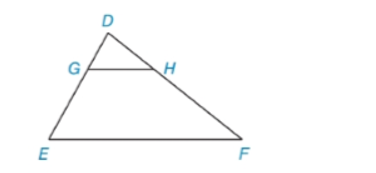Chapter 5.3, Problem 10EElementary Geometry For College St...

7th Edition
Alexander + 2 others
ISBN: 9781337614085

Solutions

Chapter
SectionElementary Geometry For College St...

7th Edition
Alexander + 2 others
ISBN: 9781337614085
Textbook Problem

In Exercises 9 to 12, name the method that explains why ∆ D G H ~ ∆ D E F . D E = 3 · D G and D F = 3 · D HTo determine

To name:

The method that explains why DGH~DEF

Explanation

Given:

The triangle,

Here,

DE=3·DG and DF=3·DH

Approach:

Two polygons are similar if and only if two conditions are satisfied.

1. All pairs of corresponding angles are congruent.

2. All pairs of corresponding sides are proportional.

Definitions:

AA:

If the two angles of one triangle are congruent to the two angles of a another triangle, then the triangles are similar.

SAS:

If an angle of one triangle is congruent to an angle of a second triangle and the pairs of sides including the angles are proportional (in length), then the triangles are similar.

SSS:

If the three sides of one triangle are proportional (in length) to the three corresponding sides of a second triangle, then the triangles are similar.

Description:

For the given triangle,

It is given that,

DE=3·DG and DF=3·DH

There are two triangles DGH and DEF

Still sussing out bartleby?

Check out a sample textbook solution.

See a sample solution

The Solution to Your Study Problems

Bartleby provides explanations to thousands of textbook problems written by our experts, many with advanced degrees!

Get Started

Solve the equations in Exercises 126. (x2+1)(x+1)43(x+1)73=0

Finite Mathematics and Applied Calculus (MindTap Course List)

In Exercises 5-8, solve for x. x2+7x=0

Calculus: An Applied Approach (MindTap Course List)

Evaluate the integrals in Problems 7-36. Check your results by differentiation. 9.

Mathematical Applications for the Management, Life, and Social Sciences

True or False: If h'(x) = k(x), then k(x) is an antiderivative of h(x).

Study Guide for Stewart's Single Variable Calculus: Early Transcendentals, 8th